# An Industrial Application Towards Classification and Optimization of Multi-Class Tile Surface Defects Based on Geometric and Wavelet Features

An Industrial Application Towards Classification and Optimization of Multi-Class Tile Surface Defects Based on Geometric and Wavelet Features

Huseyin Coskun Tuncay Yiğit* İsmail Serkan Üncü Mevlüt Ersoy Ali Topal

Computer Technologies Department, Uşak University, Uşak 64000, Turkey

Computer Engineering Department, Suleyman Demirel University, Isparta 32200, Turkey

Electrical and Electronic Engineering Department, Isparta University of Applied Sciences, Isparta 32200, Turkey

Corresponding Author Email:
tuncayyigit@sdu.edu.tr
Page:
2011-2022
|
DOI:
https://doi.org/10.18280/ts.390613
24 June 2022
|
Accepted:
1 October 2022
|
Published:
31 December 2022
| Citation

OPEN ACCESS

Abstract:

It is possible to detect visual surface defects with software in industrial tile production and increase productivity by automating the quality control process. In this process, low error rate and low cost are important indicators. In order to eliminate this negativity and the effect of the human factor, error detection software has been developed in an artificial intelligence-based industrial artificial vision environment. Spots, scratches, cracks, pore defects, which are the most common surface defects, are classified according to 6 different geometric and wavelet transform attributes. Firstly, an industrial artificial vision environment was created. In this environment, a total of 150 tile images, equal numbers from each class, were obtained on the real-time production line. The resulting images were converted into binary images by preprocessing and filtering. For classification, the support vector machines method, which performs high in two-class classifications, is used with the one versus all approach. In classifications made using RBF kernel function using wavelet features as classification performance, a higher success was achieved in all defect classes than geometric features. Real-time application software for all these processes has been developed with the Python language on Ubuntu operating system.

Keywords:

surface defects, classification, machine vision, wavelet transform, geometric features

1. Introduction

In industrial granite tile production, visual production defects such as flecks, spots, pores, cracks, scratches on tiles that are shaped by pressing and casting and on which patterns are printed after some other processes are defined as manufacturing defects. It is very important for the production quality of ceramic factories that visual production errors occur at a low level in the quality control process and can be controlled. In industrial tile production, detecting product defects and separating products according to quality classes is done with the human eye at the end of the production line. Therefore, this process is individual and depends on the experience of the employee.

The manual quality control process causes workers to feel physically tired, eyestrain, and lack of attention. This may cause products to be misclassified according to their quality class. These and similar situations may have negative consequences in terms of production costs. As a result of the manual examination, a qualitative selection is made as there is only an error or there is no error. There are many studies on this subject in the literature regarding the classification of granite tile surface defects.

Novak and Hocenski  have processed 30 industrial tiles with image quality in the 60-72 DPI range and 1142×1459 pixel dimensions. Of these, 30 have a blackhead manufacturing defect, and 30 are defect-free. In this classification, the nearest neighbor classification method based on Euclidean distance is used. In order to use it in the training set, 10 normal and faulty tile images were processed. Within the scope of the study, there was no error in classification with local binary pattern features. However, there was a 22.5% classification error according to the first level statistical coefficient attributes of the dark colored single patterned tile images. Only one defect was classified in their work.

Elbehiery et al.  tried to find crack and stain defects in ceramic tiles by using wavelet transform. Continuous, discrete, and fast wavelet transform techniques were applied to the tile images with these errors. For these techniques, using transforms covering translations up to ± 32 pixels horizontally or vertically, the 5-level wavelet coefficients of the Haar, Daubechies, Coiflets, Symlets, and Biorthogonal wavelet types were obtained. They obtained higher accuracy when they used the Daubechies wavelet type to detect the specified errors, and lower accuracy when they used the Haar wavelet type.

Najafabadi and Pourghassem  presented a system for measuring corner angles for visual inspection of ceramic tile corners. According to a method based on image processing techniques and point product vectors, the ceramic is considered defective if the corner angle is greater than 92 degrees or less than 89 degrees. The method developed in this study was evaluated on a sequence of images taken from the main system in the ceramic tile production factory and has a 12.5% error in both normal and faulty tiles.

Ghazvini et al.  by using 2D wavelet transform and statistical features, tile defects were detected. In this study, the advantages of the Haar wavelet are explored, with the recommendation to use the best fit points as the median features and to compare them with the rest of the statistical features in the wavelet domain. This method has been tested on a variety of ceramic tiles and on average applies to more than 90% of the images. Among other advantages, low computational load and high speed draw attention.

Andrade and Eduardo  provided automatic observation of ceramic tiles by means of Artificial Neural Network (ANN) method using infrared ceramic tile images. For the IR image processing system, an ANN, a system that detects defective or non-defective ceramic tiles, has been implemented. The system processes ceramic tiles in order to determine the measurement values obtained in the pattern print output. These processes have been applied to ceramic tiles containing artificial and real defects. The results obtained have proven the effectiveness of method error analysis on raw ceramic tiles and a successful approach online. It has also shown that it can be used in quality control applications.

Bianconi et al.  classified granite tiles with a computer vision system. They classified the images on 12 granite tiles with different visual properties, with 4 granite tiles in each class. It was concluded that the color and texture-based features were extremely effective. Provided that the Support Vector Machine (SVM) management parameters as a classifier are set correctly, it is seen that a better classification success is achieved than other classifiers.

Chen et al.  Machine vision methods were used to detect defects on ground patterned and patternless ceramic tile images. In this study, among 140 images, 70 images have a grinding structure and 70 images are without a pattern. In the first set of 70 images, 60 images served as training and 10 images as test samples. The same experimental procedures were carried out in the last 70 images. As the shape features, the width and height of the smallest rectangle (SR) surrounding the defect, its circumferential length, its area, the ratio of the perimeter to the area, the ratio of the defect area to the SR area, the number of pixels of the minimum convex area containing the random concave covering the defect, and the ratio of the actual defect area to this area were calculated. C4.5-based decision tree classifier was used according to shape features. As a result of this study, imperfectly ground ceramics were classified with a success rate of over 93%.

Ghita et al.  developed an automated solution based on machine vision for the identification of paint and surface defects on painted roof tiles. Local Binary Pattern (LBP) distributions are used as the texture feature, while tonal information is extracted by a self-initiated unsupervised K-means clustering algorithm. In this study, 235 tiles were tested (112 reference-perfect tiles and 123 defective tiles). The success rate for correctly classifying the tile was found to be 98.12% for perfect tiles and 99.18% for defective tiles.

Hanzaei et al.  detected glaze cracking, thin crack, pinhole, and pothole defects on the ceramic tile. In this study, all detected defects of a ceramic tile are labeled and the corresponding geometric features are extracted. The CCD camera was used to obtain the images. Of the 230 images obtained, 175 were detected with one defect, 42 with more than one defect, and 13 as perfect. Each image is 1285×1024. To characterize the defect shape, 4 different feature values were calculated extend, thinness ratio, eccentricity, and width. To characterize the defect size, 4 different geometric features as area, perimeter, main axis length, and minor axis length were obtained. Next, the one-against-all SVM classifier was used to identify the various error types. In order to obtain better classification results, Radial Basis Function (RBF) (kernel with γ=0.5) and penalty parameter C=10 is used for training all SVM classifiers. As a result of the classification, an average classification success of 94.5% was achieved, with 97% in common glaze cracks, 90.3% in fine cracks, 97% in holes and 93.7% in pits.

Macarini and Weber  propose a validation system based on image processing and machine learning for ceramic tiles. The data set consists of 783 ceramic tile images, 80% of which are training and 20% are testing. Segmentation Based Fractal Texture analysis method was used as feature extraction method. SVM is used to classify ceramic tiles. Tiles are divided into 2 classes quality and poor quality. This study reached an accuracy rate of 98.68%. In these studies, it is seen that a single feature extraction (shape, frequency, statistical) approach is used in studies using classification methods and spot, scratch, pore, fleck and crack defects, which are the subject of this study, are not examined. It also appears that very few studies are real-time, and most are offline.

This study is more than just theoretical; It offers a practical, innovative and objective system design for the surface defect control and manufacturing defect analysis using deep learning models. The traditional quality control process in industrial tile production cannot be carried out in accordance with the standards. This situation creates an important uncertainty in the control and improvement of the production process. For this reason, a system has been presented for the digitization of quality control processes based on deep learning and machine vision methods in order to produce industrial tiles in ISO standards . In addition, when the literature is reviewed, there are no studies in which all the defects examined in this study are analyzed and classified together.

In the present study, the defects of plain patterned granite tiles were tried to be classified so that the deficiency related to the subject in the literature was tried to be eliminated. First of all, a machine vision environment was created to acquire tile images. Then, a defect image set consisting of a total of 150 images from 5 different defect classes was created. The images have been filtered and binarized. Geometric features of the defects were obtained in the binary defect images. These attributes are classified by SVM. The results obtained were discussed and suggestions were made to improve the system. Application software has been developed in Python language for the real-time operation of the system.

The rest of this paper structured as follows: Section 2 is the materials & methods; the modeling of quality control processes in the ceramic tile production line and the methods of obtaining data are mentioned. Section 3 presents study results and discussion part. And the final part is, which is Section 4, Conclusion and Recommendations.

2. Material and Methodology

2.1 Machine vision environment

To obtain tile images, a machine vision environment consisting of a lighting cabinet, camera, conveyor belt, and imaging computer has been created at the laboratory and also at the factory production line of Usak Seramik. The image of the machine vision environments is given in Figure 1. An attempt was made to simulate a factory production line in the machine vision environment. Figure 1(a) is the image processing infrastructure implemented in the experimental environment. Figure 1(b) is the prototype created for the factory environment.

## 1.png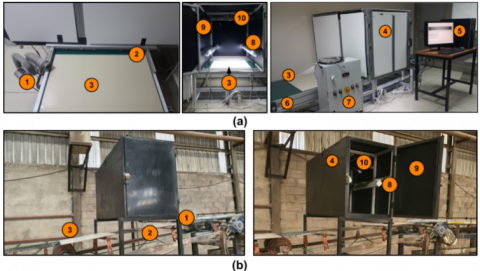Figure 1. Experimental (a) and industrial (b) machine vision environment for tile inspection

First of all, when a ceramic tile comes in line with the cabinet on the production line, the brush (2) and the air blasting machine (1) start to work. Thus, it clears the tile surface from dusts. If dusts remain on the ceramic tiles surface, the system will act as a defect, causing problems with surface quality and defect classification. At this stage, thanks to the illumination light, it provides an ideal environment that focus to attributes of the image and ensures that it is distributed homogeneously. Homogeneous distribution of light is provided on the surface of the ceramic tile, thanks to the luminaire lens and the diffuser. In this study, LED lights were preferred due to their low power consumption and small footprint. For homogeneous lighting, two linear lighting rods (8) are used at opposite angles to the surface. The green (6) conveyor belt provides controllable movement at the factory's production line speed. This speed can be changed with the speed control device (7) to test different production speeds. Thus, as the ceramic tiles (3) are inside the lighting cabinet (4), the video images of the ceramic tile are captured by the line camera (10) and sent to the software system (5) in a simple way. The inner surface of the lighting cabinet (9) is covered with matte black material to prevent reflections that may occur due to lighting. The computer in the imaging system (5) has an Intel Core i7-9750H CPU with 24GB of RAM and 12MB of cache. The graphics card is NVIDIA GeForce GTX 1050 4GB and 640 cores. Basler brand line scan camera with 51 kHz over GigaE, 2k resolution and a global shutter is used to capture the images.

The training data set of the system consists of five different defect classes: point, scratch, pore, speckle and crack. Each tile surface may have a different number and type of surface defects. 30 images were generated for crack defects. In order to create a balanced data set in the laboratory environment, 30 different defect images of each defect type (150) in 64×64 dimensions were used. Example defect images of the data set used in the study are given in Figure 2.

## 2.pngFigure 2. Defect images data set 

2.2 Filtering & binarization

The Steerable Digital Filter (SDF) acts like a Gabor filter because it has a directional structure. SDF was presented by Freeman and Adelson  in 1991. It has various applications in image processing such as texture analysis, enhancement and edge detection. In the filtering process, input data is passed through basic filters with different orientations and then subdivided to find the orientation properties. No matter what angle value of the directional filter is specified, after an image is passed through a steerable filter, the edges of that angle dominate the image, while at certain angle values, the directions away from this angle weaken or disappear completely. In order to realize this filter, the first derivative of the two-dimensional Gaussian function in Eq. (1) is applied as a steerable filter .

$g(x, y)=e^{-\left(x^2+y^2\right) / 2}$             (1)

Gaussian functions are often used to represent the probability density function of a normally distributed random variable. The normalizing constant or scaling constant is used to reduce any probability function to a probability density function with total probability of one. Since a directed filter will be applied on an image here, a probability density function with a total probability of 1 is not needed. Besides, the normalization constant is utilized for univariate Gaussians. Therefore, scaling constant can be ignored [15-17]. For this reason, the scaling constant $1/\sqrt{2} \pi$ is not taken into account in Eq. (1) for a simpler structure. A simpler example of a steerable function is presented in Eq. (2).

$g_x(x, y)=\frac{\partial g(x, y)}{\partial x}=-x e^{-\left(x^2+y^2\right) / 2}$             (2)

The orientable function can be represented with Eq. (3) in polar coordinates.

$g_x(r, \theta)=-r e^{-r^2 / 2} \cos \theta$                (3)

The expression given above results from an angular component and a radial component corresponding to a polar separable function. For example, it can be given as in Eq. (4), giving $\alpha=\pi / 2$ rotation .

$g_x\left(r, \theta-\frac{\pi}{2}\right)=-r e^{-r^2 / 2} \cos \left(\theta-\frac{\pi}{2}\right)=-r e^{-r^2 / 2} \sin (\theta)$            (4)

In fact, if the steerable function is returned to any angle α, the result can be given as Eq. (5).

$g_\alpha(r, \theta-\alpha)=\cos (\alpha) g_x+\sin (\alpha) g_y$            (5)

where, gx and gy denote horizontally and vertically oriented derivatives in Eq. (5), respectively. To use the SDF on an image, the orientation properties of the input image must be revealed. The image used as input is first passed through basic filters with different orientations and then subdivided. For this reason, a filter function with a rotatable structure in Eq. (6) can be given as a linear sum of its rotated versions.

$g_\alpha(x, y)=\sum_{i=1}^M k_j(\alpha) g_{\alpha j}(x, y)$           (6)

The resulting image consists of certain directional impact responses for the system defined at a certain angle in the α value and the weighted sum of gαj(x, y) in the α direction. kj(α) are the weight coefficients and provide control of the filter orientation in the α direction.

For the SDF filter, the directed filter responses of the defect images are processed as a filter output image. Pixel values indicating edges in different directions and in the same direction are converted to binary images in binaryization with the image used as output. First, the filter output matrix is subtracted numerically from the grayscale defect images matrix. The average values of the filter output image are then obtained as a one-dimensional array. Then the average value of the average one-dimensional array is calculated. Pixel values greater than the threshold value in the faulty image sequence are obtained from binary output images. In this way, the adaptive thresholding method is realized. Binarization process is given in Figure 3.

In line scanning cameras, resolution and image transfer rate are calculated according to the speed of the moving object, unlike area scanning cameras. In this context, the required transmission rate and camera line frequency rate to obtain tile images were calculated. By using the desired image width (W), the number of pixels per mm (ppm) and the conveyor belt speed (S), the image size to be transferred per second from the line scan camera was calculated in MB/s. The number of pixels corresponding to the real area of 100 mm2 on the image were measured horizontally and vertically using the ImageJ program. By taking the average of these values, the average pixel number (ppm) corresponding to 1 mm was calculated. The measurement steps are given in Figure 4. The 100 mm2 area width was measured as 32 pixels and the height as 31 pixels by the camera. The mean ppm value was calculated as 3.15 and measured as 100 mm2, 99.975 mm2, and 992 pixels. The relative error was found to be 0.00025. Since the relative error is quite small, it is considered negligible.

## 3.png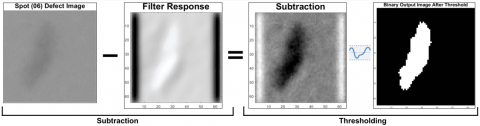Figure 3. Binarization process

## 4.png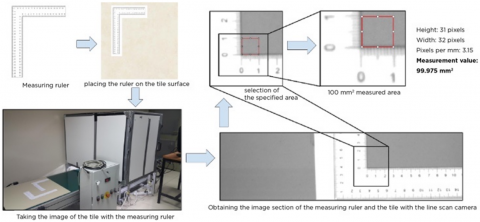Figure 4. Calculation of the average number of pixels for 1mm

## 5.png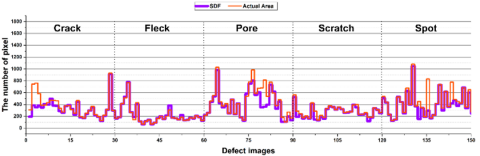Figure 5. Number of defect area pixels calculated after filtering

## 6.png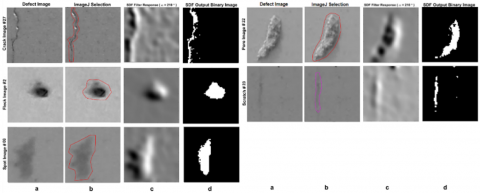Figure 6. Results of filtering and binarization, (a) The raw surface defect image, (b) The actual defect area pixels in ImageJ program, (c) SDF filter response, (f) SDF output binary image

## 7.pngFigure 7. Segmentation and feature extraction process

The ability to represent real defect areas of binary images is demonstrated by comparing the pixel counts of the real defect areas with the pixel counts of the binary images. The number of pixels formed in the output images is counted after binaryization. The actual area pixel number is calculated manually by the ceramic engineers at Uşak Seramik via the ImageJ program and is accepted as a reference value. SDF filtering images of the randomly selected error image from the error classes, binary images, and the actual error area selection obtained using ImageJ are given in Figure 5.

The number of defect area pixels of the defect images in the dataset was found and given in Figure 6. It was observed that the number of defective area pixels calculated using the SDF matched the actual number of defective areas in Figure 6.

2.3 Feature extraction

Geometric Feature: Before debugging the features of the errors, the image areas of the errors in the filtered and preprocessed tile image segment should be segmented. Black vertical lines and horizontal lines in the section image are removed for segmentation. The remaining white areas are defined as defective areas. To calculate the geometric features, specific features of the defect must be determined. Geometric features were determined from the pixel value positions (coordinates) on the binary defect image. The segmentation and feature extraction process of the defect images on the sample tile image section containing the spot defect in the software environment is given in Figure 7.

Explanations of the geometrical properties specified in Figure 6 are given in Table 1. The h is explained as the height of the smallest rectangle surrounding the defect and calculated as the number of pixels along the defect length. The w is explained as the width of the smallest rectangle surrounding the defect and calculated as the number of pixels across the width of the defect.

Table 1. Geometric properties of defect

 Symbol Geometric feature Description h Height of the smallest rectangle surrounding the defect The number of pixels along the defect length. w The width of the smallest rectangle surrounding the defect The number of pixels across the width of the defect. m Major axis length of smallest ellipse surrounding the defect (longest axis) The distance from the last pixel of the first pixel representing the defect horizontally along the defect length n Minor axis length (shortest axis) of the smallest ellipse surrounding the defect The distance from the last pixel of the first pixel representing the defect vertically across the defect width F Each focal point of the smallest ellipse surrounding the defect The distances of the focal points from the center of the smallest ellipse representing the defect $F^2=\left(\frac{m}{2}\right)^2-\left(\frac{n}{2}\right)^2, f=2 F$ a The distance from the focal point of the smallest ellipse surrounding the defect to the lateral axis end point The distance of the first pixel representing the defect horizontally along the defect length to the last pixel representing the defect vertically along the defect width

Table 2. Geometric features of defect

 Feature Calculation value or equation Description A1 Defect area $\mathrm{A}=\sum_{r=0}^{h-1} \sum_{c=0}^{w-1} g(r, c)$ The area is defined as the total number of pixels attached to the domain of any detected defect target (gi), with r number of rows and c number of columns . A2 Area of the smallest rectangle surrounding the defect $w * h$ P1 Perimeter of defect $P=\sum \begin{gathered}g_i(r, c)=1 \\ \text { if } g_i(r+1, c) \\ \text { or } g_i(r-1, c) \\ \text { or } g_i(r, c+1) \\ \text { or } g_i(r, c-1)=0\end{gathered}$ This is the number of pixels on the edge of the defective area. Represents the number of white pixels surrounded by a black pixel to obtain the perimeter of the defective area. P2 Perimeter of the smallest rectangle surrounding the defect $2 *(w+h)$ F1 Extend ratio $\mathrm{A}_1 / A_2$ Dividing the area of the defect by the area of the smallest rectangle surrounding the defect F2 Lining ratio $P_1 / P_2$ Dividing the defect perimeter by the perimeter of the smallest rectangle surrounding the defect

## 8.png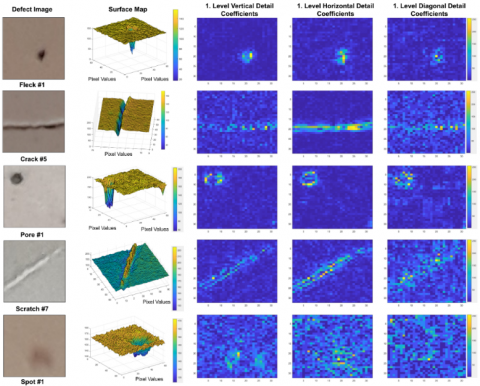Figure 8. Vertical, horizontal and diagonal detail coefficients of sample defect images

The geometric features are given in Table 2 were calculated by using the geometric properties specified in Figure 7 and Table 1.

Wavelet Features: The wavelet transform method is used to extract the frequency domain features of the defect images. Wavelet transform is a feature extraction tool that divides data, functions, or operators into different frequency components and then examines each component with a resolution appropriate to its scale . The wavelet transform uses narrower windows as window length at high frequencies, while wider windows are used at low frequencies. Therefore, it is useful in the frequency domain when converting a signal or image into lower-dimensional data that can be represented. For this purpose, a single-level 2-dimensional (2-D) Haar-type discrete wavelet transform was used for images. First, a 1-D filter bank was applied to the rows of the image. Then, the same transformation is applied to the columns of each channel of the result. For this reason, 3 high-pass channels (detail coefficient) corresponding to vertical, horizontal, and diagonal and one approach image were obtained. The resulting surface view map of the vertical, horizontal, and diagonal 1st level detail coefficients is presented in Figure 8. The averages of the obtained wavelet coefficients were calculated and evaluated as the feature value. The mean, variance, and standard deviations of the obtained wavelet coefficients were calculated, respectively. These values are evaluated as the feature value. The best accuracy result has been obtained through diagonal coefficients.

2.4 SVM classification

As a machine learning algorithm, the SVM based on supervised learning, which is used as a fast classifier in the literature, was used. In simple terms, SVM can be defined as the separation of the data of two different classes on the same plane, with a boundary line formed by a linear equation. SVM is a kernel-based learning approach that gives very fast and accurate results in subjects such as linear and nonlinear classification, regression analysis, outlier detection, function, and density estimation. It is especially used in classification problems where the patterns between the variables of the data to be classified in data mining are not known. Real-life classification problems are often non-linear problems made up of many different classes. SVM aims to solve these problems more easily by making the nonlinear sample space linear on a higher plane. While doing this, it tries to find the linear equation that maximizes the distance between the closest elements of the classes. This is called margin maximization in the literature [19-23]. In other words, SVM aims to create the furthest upper (hyper) plane between the vectors of two classes with a linear decision function. The positive aspects of SVM can be listed as follows;

• It does not require prior knowledge assumption about the distribution.

• It achieves high accuracy values.

• Model complex decision boundaries.

• It can work with a large number of independent variables.

• It can work with both linear and non-linearly separable data.

• Compared to other classification methods, the problem of overfitting is less.

The SVM method is widely used in two-class problems .

Within the scope of the study, the data processed in SVM were used linearly. The problem that is tried to be solved in this paper is accepted as a multiple classification problem because it consists of 5 classes. SVM is insufficient as it stands. For this reason, for each class belonging to the surface defect classes, it was tried to be solved as a binary classification problem by accepting all other classes as a single class. It has been accepted as the one against all (one vs. all) method in the literature. Two feature types should be used in the solution of binary classification problems. Since the best results from these features were obtained from F1 and F2 features. These features were used for classification. Of the 150 feature values, 90 data (60%) were used for training and 60 data (40%) for test. Polynomial and RBF are used as SVM kernel functions. The Sequential Minimum Optimization (SMO) method was chosen as the optimization solver in the SVM model. In the studies conducted with the SVM algorithm, the effect of different kernel functions on classification success was investigated. In some studies, the RBF kernel function-based classifier was found to be more successful, and in some studies, the polynomial kernel-function classifier was more successful [25-30]. Therefore, in this study, the success of both core functions in classification was measured.

3. Metrics

The confusion matrix and evaluation criteria were used to measure classification success. Since there is more than one classification in this study, a complexity matrix was obtained for the performance measurement for each defect class. For this reason, a complexity matrix was created for each class according to the definition of actual values and predicted values in Table 3.

Table 3. Confusion matrix definition of each class

 Prediction Reference Defect Class Other Classes Defect Class True Positive (TP) False Positive (FP) Other Classes False Negative (FN) True Negative (TN)

Table 4. Classification evaluation metrics

 Metric Formula Accuracy (A) $\frac{t p+t n}{t p+t n+f p+f n}$ Precision (P) $\frac{t p}{t p+f p}$ Recall (Sensitivity) (R) $\frac{t p}{t p+f n}$ F-Score $\frac{2\left(\frac{t p}{t p+f n}\right)\left(\frac{t p}{t p+f p}\right)}{\left(\frac{t p}{t p+f n}\right)+\left(\frac{t p}{t p+f p}\right)}$ Specificity (S) $\frac{t n}{f p+t n}$ AUC $\frac{1}{2}\left(\frac{t p}{t p+f n}+\frac{t n}{t n+f p}\right)$ False Positive Rate (FPR) $\frac{f p}{f p+t n}$ Balanced Accuracy (BA) $\frac{1}{2}\left(\frac{t p}{t p+f p}+\frac{t n}{f p+t n}\right)$

A confusion matrix with a multi-class structure was used to measure the performance of each class, such as Precision, Sensitivity, Accuracy, Specificity, F-Score, Balanced Accuracy, AUC (Area Under the Curve), and False Positive Ratio. The formulas of these measurement metrics are given in Table 4.

For the implementation of the methods described, the application software was developed in Python (3.8) and the Keras (Quasi-SVM) library was used for SVM. The application software has been tested on the Ubuntu (21.04) operating system.

4. Results

The classification was carried out by selecting the F1- Extend ratio and F2- Lining ratio feature groups using the SVM method. F1 and F2 features data graphics are given in Figure 9.

1st level wavelet detail coefficients of vertical, horizontal, and diagonal are given in Figure 10 respectively.

Classification results of geometric and wavelet feature with different kernel functions (RBF, polynomial) are given in Figure 11a, Figure 11b, Figure 11c, and Figure 11d respectively.

ROC (Receiver Operator Characteristic) graphics of each defect class of geometric features with different kernel functions (RBF, polynomial) are given in Figure 12a and Figure 12b respectively. ROC (Receiver Operator Characteristic) graphics of each defect class of wavelet features with different kernel functions (RBF, polynomial) are given in Figure 12c and Figure 12d respectively.

The confusion matrix of each class of geometric and wavelet features with RBF and polynomial kernel function are given in Figure 13a, Figure 13b, Figure 13c, and Figure 13d respectively. The classification performance results obtained using the confusion matrix are given in Table 5.

When Table 5 is examined, it is seen that 100% classification success is achieved for all classes and all core functions with wavelet features. It is seen that a higher success value is obtained compared to the results obtained in the previous study  and manual examination. Also, it seems that kernel functions do not have a direct effect on classification success. The validation losses for the cross-validation classification model of the classification model obtained using the SVM method are given in Table 6.

It can be seen that the validation loss is quite low for the attributes and kernel functions of all classes from Table 6. This result shows that correct results are produced for classification with SVM in all feature groups. Although it is understood that there is no effect of kernel functions in the classification results given in Figure 12c, Figure 12d, Figure 13c, Figure 13-d, and Table 5 for the wavelet feature group, when the validation loss values given in Table 6 are examined, it is more difficult because the validation loss of the radial basis kernel function is small when the data set grows. It is expected to produce successful results. The real-time application interface of detection system is given in Figure 14. The localizations of the defects are also determined in real-time application. The application software is developed in Python language. It is planned to run on operating systems such as Windows, Ubuntu. Also, it can run computers such as Raspberry Pi or Nvidia Jetson tx2. The developed system was set up for testing at the Uşak Seramik factory. The industrial machine vision environment is given in Figure 15.

## 9.png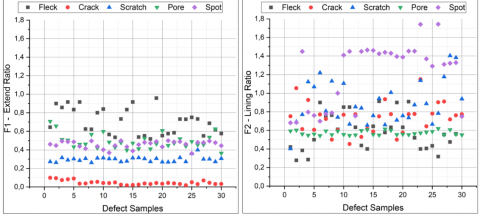Figure 9. F1 and F2 feature set

## 10.png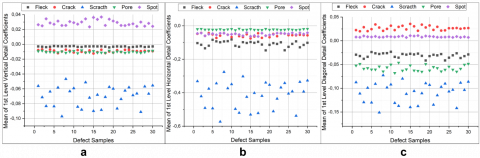Figure 10. 1st level wavelet detail coefficient means, (a) vertical, (b) horizontal, (c) diagonal

## 11.png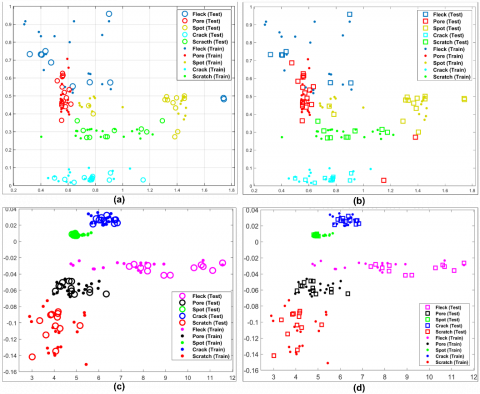Figure 11. Classification results

## 12.pngFigure 12. ROC area of each class

## 13.png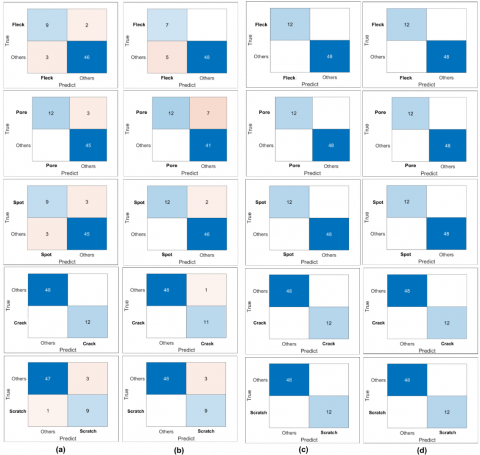Figure 13. Confusion matrix of each class

Table 5. Classification results

 Defect Feature Kernel A P R F S BA AUC FPR Fleck Geo R 0.91 0.81 0.75 0.78 0.95 0.88 0.85 0.041 P 0.91 1 0.58 0.73 1 1 0.79 0 Wavelet R 1 1 1 1 1 1 1 1 P 1 1 1 1 1 1 1 1 Pore Geo R 0.95 0.8 1 0.88 0.93 0.86 0.96 0.06 P 0.88 0.63 1 0.77 0.8 0.85 0.92 0.14 Wavelet R 1 1 1 1 1 1 1 1 P 1 1 1 1 1 1 1 1 Spot Geo R 0.9 0.75 0.75 0.75 0.93 0.84 0.84 0.06 P 0.96 0.85 1 0.92 0.95 0.95 0.97 0.041 Wavelet R 1 1 1 1 1 1 1 1 P 1 1 1 1 1 1 1 1 Crack Geo R 1 1 1 1 1 1 1 0 P 0.98 1 0.91 0.95 1 1 0.95 0 Wavelet R 1 1 1 1 1 1 1 1 P 1 1 1 1 1 1 1 1 Scratch Geo R 0.93 0.9 0.75 0.81 0.97 0.93 0.86 0.02 P 0.95 1 0.75 0.85 1 1 0.87 0 Wavelet R 1 1 1 1 1 1 1 1 P 1 1 1 1 1 1 1 1 Note: R: RBF Kernel, P: Polynomial Kernel, A: Accuracy, P: Precision, R: Recall, F: F-Score, S: Specificity, BA: Balanced Accuracy, FPR: False Positive Rate

Table 6. Validation loss for the cross-validation classification model of classes with the SVM

 Features Kernel Fleck Pore Spot Crack Scratch Geometric R 0.066 0.022 0.033 0 0.011 P 0.0055 0.099 0.022 0 0.022 Wavelet R 0.066 0 0 0 0.011 P 0.055 0.022 0.033 0.011 0.011

## 14.pngFigure 14. Real-time application interface

## 15.jpg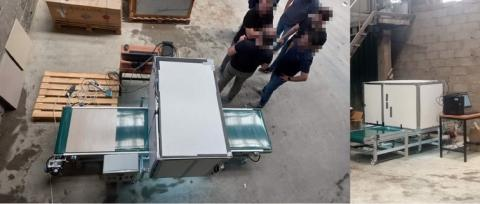Figure 15. Test phase of industrial machine vision environment

5. Conclusion and Future Works

In this study, a real-time application has been developed to classify defects such as cracks, flecks, pores, scratches, spots on the surface of granite tiles. First, the defect image dataset was created. SDF filtering and binarization processes were performed to make the defect areas clear. The defect images were obtained as a result of segmentation from the tile image sections obtained from the line scan camera. The geometric features such as extend ratio and lining ratio of defects were obtained. Within the scope of the multiple classification problem, it has been classified using the SVM classifier (one vs. all) approach. The effect of different kernel functions on classification success was researched. With the RBF kernel function, pore and crack defects were classified more successfully, and with the polynomial kernel function, spot and scratch defects were classified more successfully. In general, classification successes for different kernel functions are quite close to each other. As a result, a high-performance real-time defect detection software has been developed. The defect area detection capabilities of different filtering methods are comparable to SDF. The results of this study can be compared by classifying the defect images in the dataset with different classification algorithms.

Acknowledgment

This study, which has the Project Number TEYDEB-5190086, received support from the Scientific and Technological Research Council of Turkey (TUBITAK). We would like to thank Uşak Seramik for allowing the use of its own tiles in order to detect the actual defect of the industrial tiles and to the anonymous referees who contributed to the improvement of the work carried out thanks to their comments.

References

 Novak, I., Hocenski, Z. (2005). Texture feature extraction for a visual inspection of ceramic tiles. In Proceedings of the IEEE International Symposium on Industrial Electronics, ISIE 2005, pp. 1279-1283. https://doi.org/10.1109/ISIE.2005.1529109

 Elbehiery, H.M., Hefnawy, A.A., Elewa, M.T. (2008). Visual inspection for fired ceramic tile’s surface defects using wavelet analysis. In proceedings of ICCES’08, pp. 1187-1198.

 Najafabadi, F.S., Pourghassem, H. (2011). Corner defect detection based on dot product in ceramic tile images. In 2011 IEEE 7th International Colloquium on Signal Processing and Its Applications, Penang, Malaysia, pp. 293-297. https://doi.org/10.1109/CSPA.2011.5759890

 Ghazvini, M., Monadjemi, S.A., Movahhedinia, N., Jamshidi, K. (2009). Defect detection of tiles using 2D-wavelet transform and statistical features. World Academy of Science, Engineering and Technology, 37: 901-904. https://doi.org/10.5281/zenodo.1328348

 Andrade, R.M.D., Eduardo, A.C. (2011). Methodology for automatic process of the fired ceramic tile's internal defect using IR images and artificial neural network. Journal of the Brazilian Society of Mechanical Sciences and Engineering, 33: 67-73. https://doi.org/10.1590/S1678-58782011000100010

 Bianconi, F., González, E., Fernández, A., Saetta, S.A. (2012). Automatic classification of granite tiles through colour and texture features. Expert Systems with Applications, 39(12): 11212-11218. https://doi.org/10.1016/J.ESWA.2012.03.052

 Chen, S., Lin, B., Han, X., Liang, X. (2013). Automated inspection of engineering ceramic grinding surface damage based on image recognition. The International Journal of Advanced Manufacturing Technology, 66(1): 431-443. https://doi.org/10.1007/S00170-012-4338-2

 Ghita, O., Whelan, P.F., Carew, T., Padmapriya, N. (2005). Quality grading of painted slates using texture analysis. Computers in Industry, 56(8-9): 802-815. https://doi.org/10.1016/J.COMPIND.2005.05.008

 Hanzaei, S.H., Afshar, A., Barazandeh, F. (2017). Automatic detection and classification of the ceramic tiles’ surface defects. Pattern Recognition, 66: 174-189. https://doi.org/10.1016/j.patcog.2016.11.021

 Macarini, L.A., Weber, T.O. (2017). Quality Control System for Ceramic Tiles using Segmentation-based Fractal Texture Analysis and SVM. Sibgrapi2017.

 https://www.iso.org/obp/ui/#iso:std:iso:9001:ed-5:v1:en/

 Coskun, H., Yi̇ği̇t, T., Üncü, İ.S. (2022). Integration of digital quality control for intelligent manufacturing of industrial ceramic tiles. Ceramics International, 48(23A): 34210-34233. https://doi.org/10.1016/j.ceramint.2022.05.224

 Freeman, W.T., Adelson, E.H. (1991). The design and use of steerable filters. IEEE Transactions on Pattern Analysis and Machine Intelligence, 13(9): 891-906. http://dx.doi.org/10.1109/34.93808

 Giron-Sierra, J.M. (2017). Digital signal processing with matlab examples. Singapore: Springer Singapore.

 Chen, L.H., Goodman, T.N., Lee, S.L. (2004). Asymptotic normality of scaling functions. SIAM Journal on Mathematical Analysis, 36(1): 323-346. https://doi.org/10.1137/S0036141002406229

 Bromiley, P. (2003). Products and convolutions of Gaussian probability density functions. Tina Memo, No. 2003-003.

 Murphy, K.P. (2012). Machine learning: a probabilistic perspective. MIT press.

 Avcı, D., Sert, E. (2021). An effective Turkey marble classification system: Convolutional neural network with genetic algorithm-wavelet kernel-Extreme learning machine. Traitement du Signal, 38(4): 1229-1235. https://doi.org/10.18280/ts.380434

 Suwais, K., Alheeti, K., Al_Dosary, D. (2022). A review on classification methods for plants leaves recognition. International Journal of Advanced Computer Science and Applications, 13(2): 92-100. https://doi.org/10.14569/IJACSA.2022.0130211

 Angulo, C., Ruiz, F.J., González, L., Ortega, J.A. (2006). Multi-classification by using tri-class SVM. Neural Processing Letters, 23(1): 89-101. https://doi.org/10.1007/S11063-005-3500-3

 Guo, H., Wang, W. (2015). An active learning-based SVM multi-class classification model. Pattern Recognition, 48(5): 1577-1597. https://doi.org/10.1016/J.PATCOG.2014.12.009

 Hou, Z., Parker, J.M. (2005). Texture defect detection using support vector machines with adaptive Gabor wavelet features. In 2005 Seventh IEEE Workshops on Applications of Computer Vision (WACV/MOTION'05), Breckenridge, CO, USA, 275-280. https://doi.org/10.1109/ACVMOT.2005.115

 Malarvel, M., Singh, H. (2021). An autonomous technique for weld defects detection and classification using multi-class support vector machine in X-radiography image. Optik, 231: 166342. https://doi.org/10.1016/J.IJLEO.2021.166342

 Han, H., Jiang, X. (2014). Overcome support vector machine diagnosis overfitting. Cancer Informatics, 13: CIN-S13875.

 Boudiaf, A., Benlahmidi, S., Harrar, K., Zaghdoudi, R. (2022). Classification of surface defects on steel strip images using convolution neural network and support vector machine. Journal of Failure Analysis and Prevention, 22(2): 531-541. https://doi.org/10.1007/S11668-022-01344-6

 Jian, Z., Wei, Z. (2010). Support vector machine for recognition of cucumber leaf diseases. In 2010 2nd International Conference on Advanced Computer Control, Shenyang, China, pp. 264-266. https://doi.org/10.1109/ICACC.2010.5487242

 Li, Y., Chang, J., Tian, Y. (2022). Improved cost-sensitive multikernel learning support vector machine algorithm based on particle swarm optimization in pulmonary nodule recognition. Soft Computing, 26(7): 3369-3383. https://doi.org/10.1007/S00500-021-06718-W/FIGURES/5

 Yalsavar, M., Karimaghaee, P., Sheikh-Akbari, A., Khooban, M.H., Dehmeshki, J., Al-Majeed, S. (2022). Kernel parameter optimization for support vector machine based on sliding mode control. IEEE Access, 10: 17003-17017. https://doi.org/10.1109/ACCESS.2022.3150001

 Kavzoglu, T., Colkesen, I. (2009). A kernel functions analysis for support vector machines for land cover classification. International Journal of Applied Earth Observation and Geoinformation, 11(5): 352-359. https://doi.org/10.1016/J.JAG.2009.06.002

 Ur Rehman, H., Anwar, S., Tufail, M. (2020). Machine vision based plant disease classification through leaf imagining. Ingenierie Des Systemes d'Information, 25(4): 437-444. https://doi.org/10.18280/isi.250405